# Flipping and Reflecting in Graphing Modified Functions

Go back to  'Functions'

In this section, we will discuss graphs and graph-plotting in more detail. Detailed graph plotting also requires a knowledge of derivatives. Here, we will be discussing some general issues related to graphs. Graphs will be discussed again later in the section on differential calculus.

Up till now, we have discussed some standard functions and their graphs. We have also plotted graphs for other functions that are some variants of the standard functions.

Lets formalise all that discussion here. Given the graph of $$y = f (x),$$ how will you draw the graphs of

 (a)   $$y = - f\left( x \right)$$ (b)   $$y = f\left( { - x} \right)$$ (c)   $$y = \left| {f\left( x \right)} \right|$$ (d)   $$y = f\left( {\left| x \right|} \right)$$ (e)   $$\left| y \right| = f\left( x \right)$$ (f)   $$y = f\left( x \right) \pm k$$ (g)   $$y = f\left( {x \pm k} \right)$$ (h)   $$y = kf\left( x \right)$$ (i)   $$y = f\left( {kx} \right)$$

[a]      $$\boxed{y = f\left( x \right)\,\,{\text{to}}\,\,y = - f\left( x \right)\,}$$

This part is the easiest. We just flip the graph about the $$x\text{-axis}$$ so that positive values become negative and negative become positive.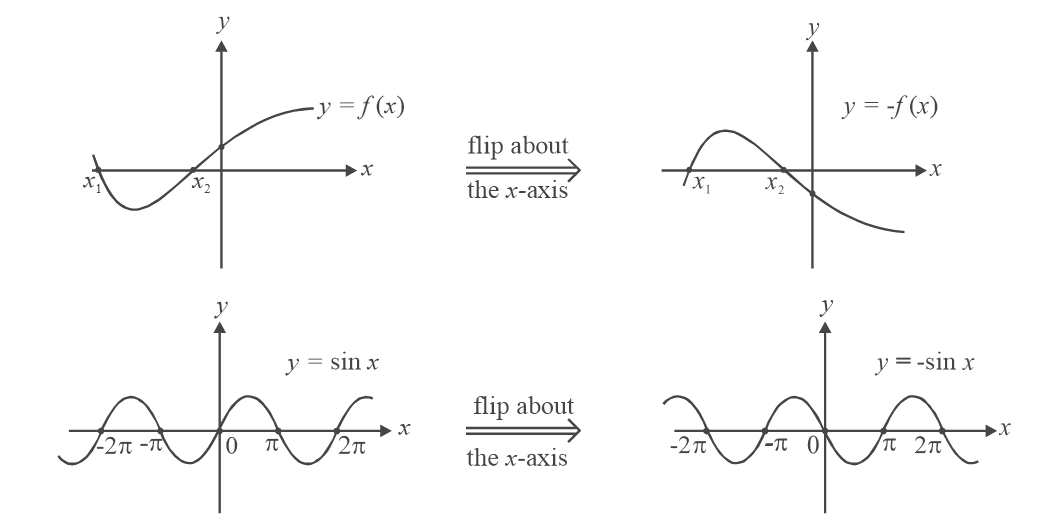[b]     $$\boxed{y = f\left( x \right)\,\,{\text{to}}\,\,y = f\left( { - x} \right)\,}$$

To draw $$y=–f(x) \text{ from } y=f(x),$$ we flipped our original graph about the $$x\text{–axis.}$$ What should we do to draw the graph of $$y=f(–x)?$$ Flip the graph about the $$y\text{–axis.}$$ This is obvious once you realise that any value of $$y$$ that was initially associated with $$x (\text{i.e } y = f (x))$$ will now be associated with $$-x, \;(\text{i.e } y = f (– (–x)).$$ For example consider $$y=x^3.$$ At $$x = 2,\; y = 8.$$ In $$y={(-x)^3},$$ what value of $$x$$ gives $$y=8?$$ Obviously, $$x = –2$$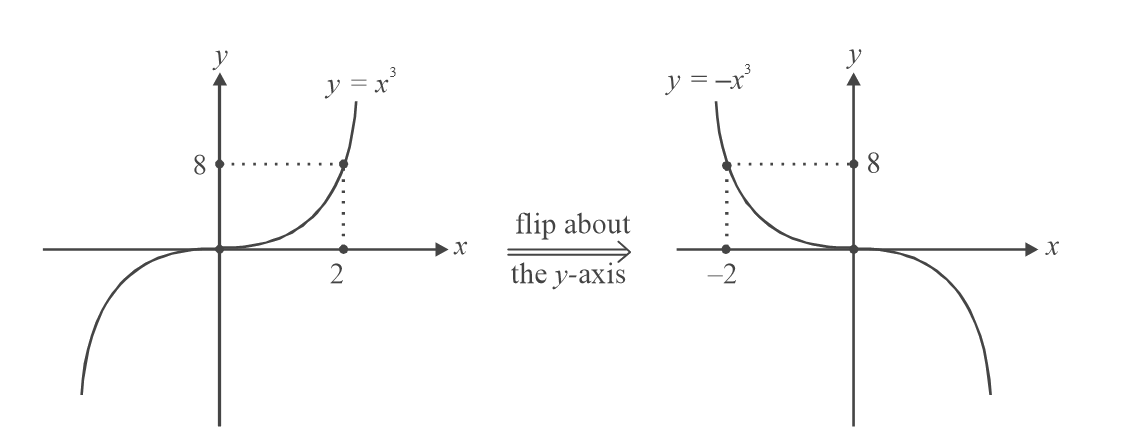Note that in this particular case, $$y={(-x)^3},$$ can also be obtained as in part [a], that is, by flipping the graphs about the $$x\text{-axis.}$$ This is because $$- {(x)^3}\,\,{\rm{and }}{( - x)^3}$$ are the same.

[c]     $$\boxed{y = f\left( {x\,} \right)\,\,{\text{to}}\,\,y = \left| {f\left( x \right)} \right|}$$

The modulus function gives the magnitude of its argument (and returns a positive output). In other words, wherever the graph of $$f(x)$$ lies below the $$x\text{-axis}$$ (that is, wherever $$f(x)$$ is negative), the modulus function will make it positive), of exactly the same magnitude, or put differently, will take a reflection of the negative part of $$f(x)$$ into the upper half of the axes.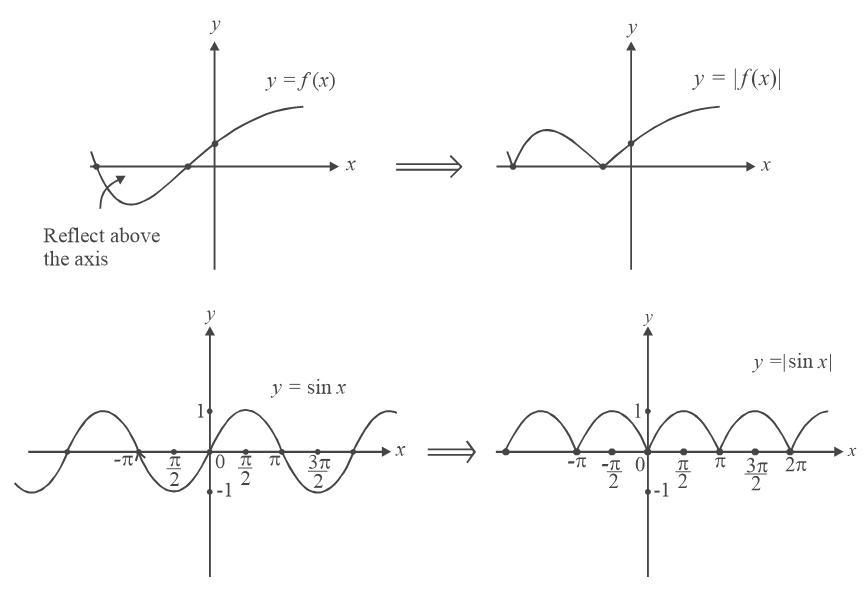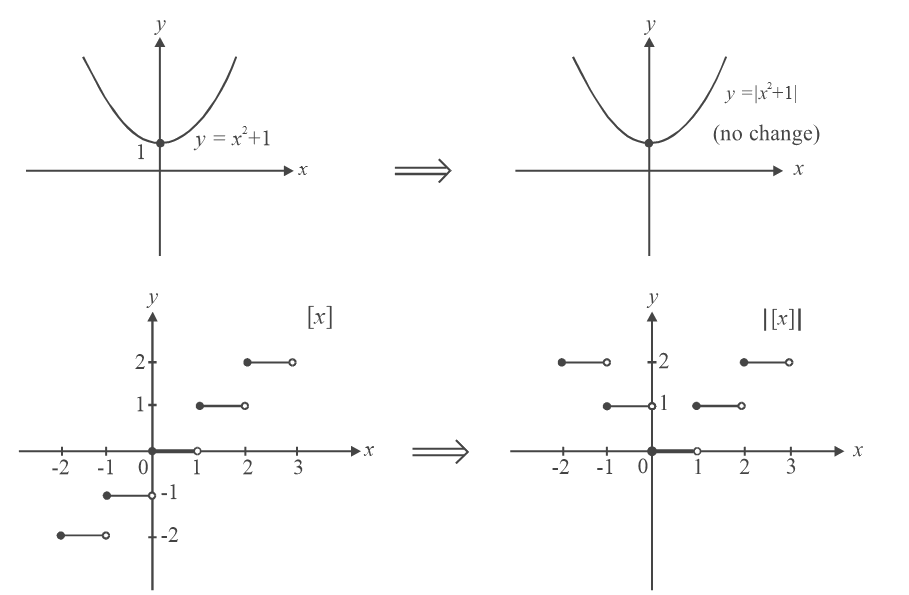[d]     $$\boxed{y = f\left( x \right)\,{\text{to}}\,y = f\left( {\left| x \right|} \right)}$$

Consider the equation $$\,y = f\left( {\left| x \right|} \right)$$  carefully. In this equation, the input to $$f$$ is only positive. (Even if you input negative values for $$x,$$ the modulus function reduces it to a positive value.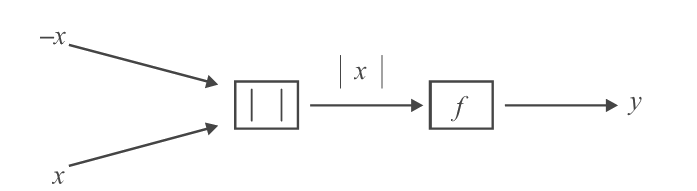Also, we see that the input $$(x)$$ and $$(–x)$$ give the same output. How should we obtain the graph? In the graph of $$y = f (x),$$ first discard the part that lies to the left of the $$y\text{-axis.}$$ This part of the graph has no use now since the input to $$f$$ (argument of $$f,$$ or the independent variable) takes only positive values. After discarding this left part, take the reflection of the right side of the graph into the left side. (Because the output of $$f$$ is same, whether the input is $$x$$ or $$–x.$$) The examples below will make it clear: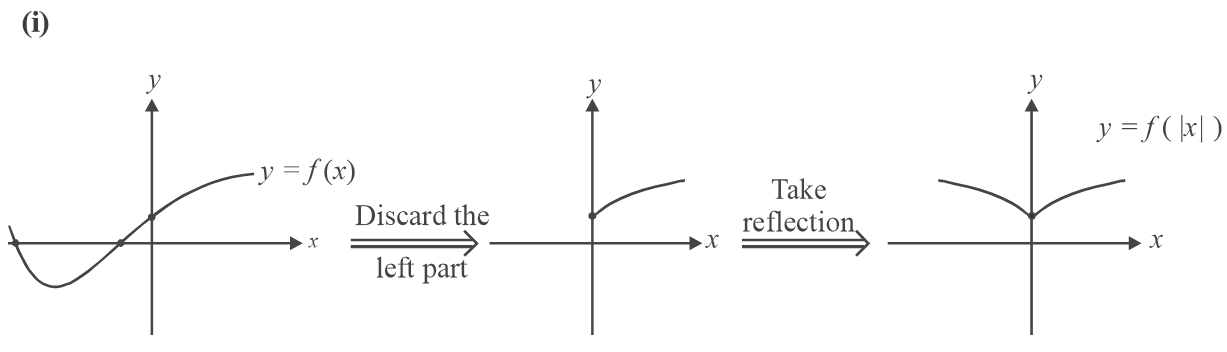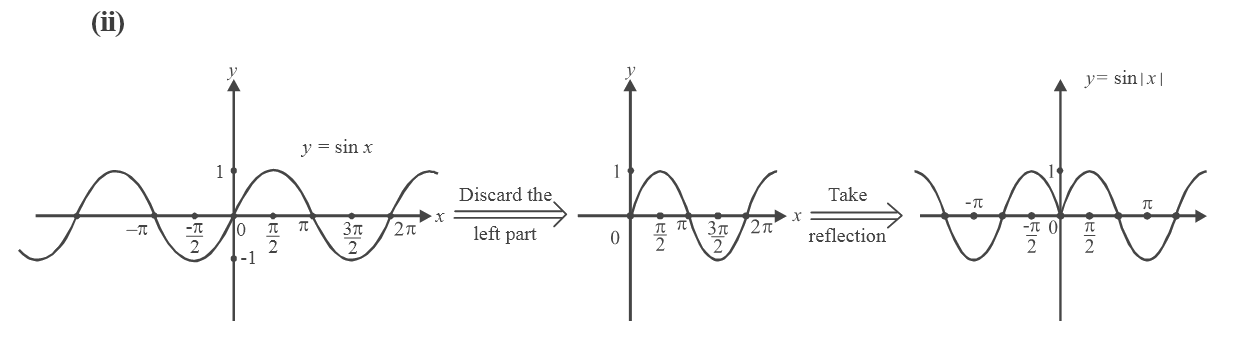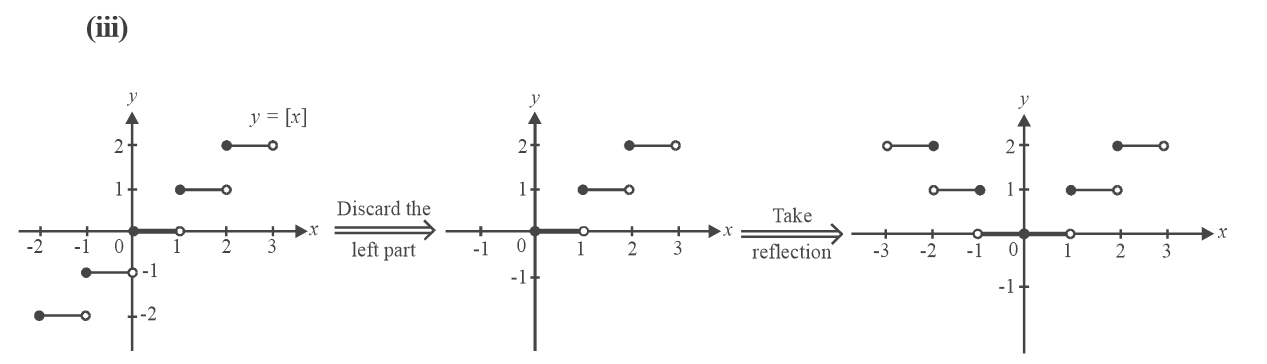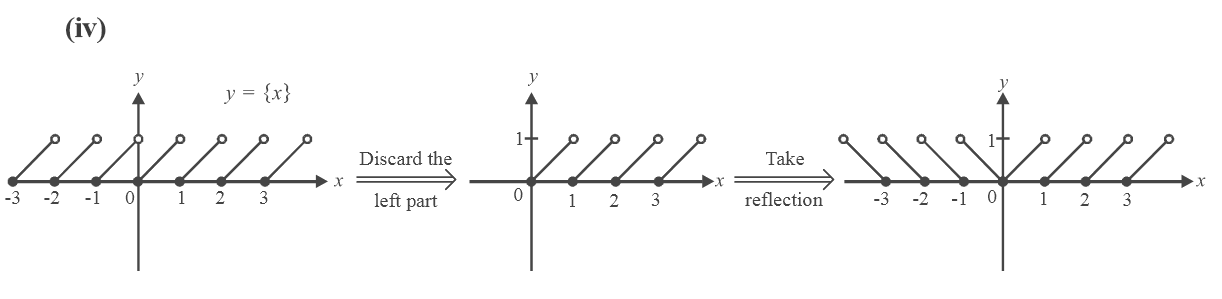[e]     $$\boxed{y = f\left( x \right)\,{\text{to}}\,\left| y \right| = f\left( x \right)}$$

If you’ve understood the case for   $$y = f\,\left( {\left| x \right|} \right)$$ , this should not be very hard. You can approach this problem in any of the following ways:

• In drawing $$y = f\,\left( {\left| x \right|} \right)$$ , we discarded the left part of the graph $$y = f (x)$$ and took a reflection of the right part into the left part. Here, the modulus function is on $$y.$$ What should we do? Discard the lower part and take the reflection of the upper part into the lower half of the axes.

• In the equation $$\left| y \right| = f\left( x \right),$$ the LHS is non-negative, so $$f (x)$$ necessarily needs to be non-negative. Hence, wherever $$f (x)$$ becomes negative, we have to discard that part, that is, we have to discard that part of the graph that lies below the x-axis. Also, if $$(x{_1},{\rm{ }}y{_1})$$ satisfies the equation $$\left| y \right| = f\left( x \right),$$ $$(x{_1}, {\rm{ }}-y{_1})$$ also satisfies this equation, or in other words, if  $$(x{_1},{\rm{ }}y{_1})$$ lies on the graph of $$\left| y \right| = f\left( x \right),$$ $$(x{_1},{\rm{ }}-y{_1})$$ will also lie on this graph, or the graph will be symmetrical about the $$x{\text{-axis}}{\text{.}}$$ Therefore, to obtain the graph of $$\left| y \right| = f\left( x \right)$$  from $$y = f (x),$$ we discard its lower part and take a reflection of the upper part into the lower half of the axes.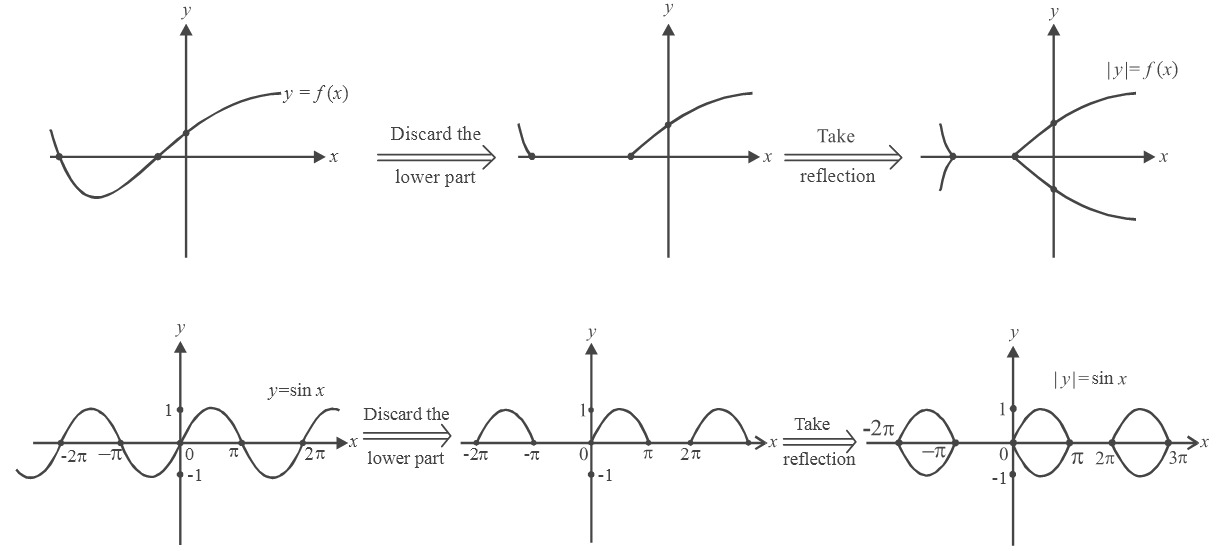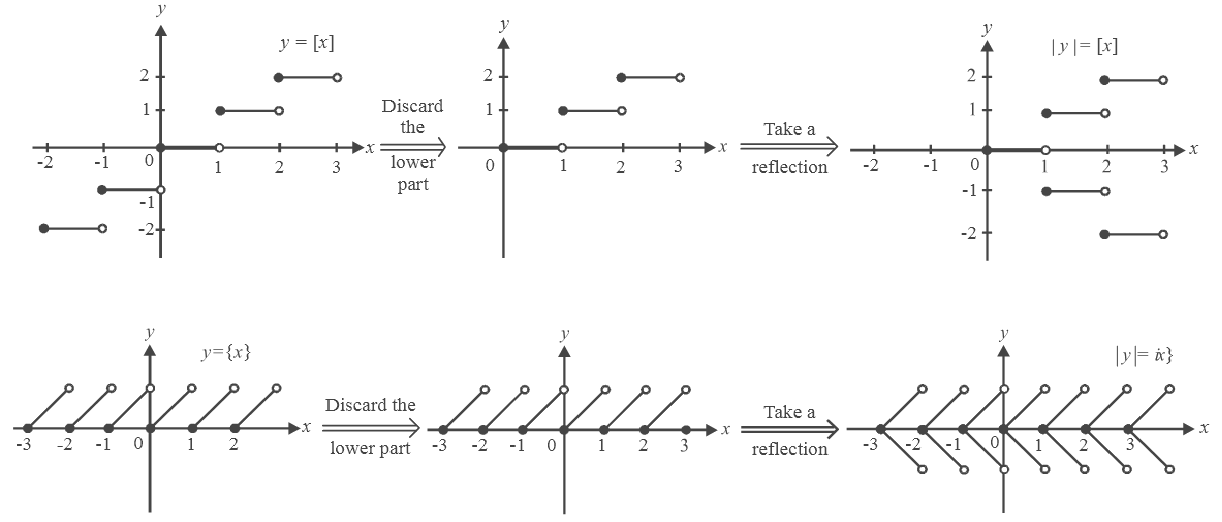Note that the equation $$\left| y \right| = f\left( x \right)$$ does not represent a function since it becomes one-many. It represents a dependence between $$x\;{\text{and}}\;y.$$